# Selina Solutions Concise Maths Class 10 Chapter 15 Similarity (With Applications to Maps and Models) Exercise 15(E)

This exercise contains problems based on all the concepts discussed in this Chapter. Moreover, this exercise concludes the Chapter with different models of problems. The Selina Solutions for Class 10 Maths is the right resource for every student who is looking to improve his/her ability of solving problems and to get a strong grip over the concepts of ICSE Class 10 Maths. For a better understanding of the concepts, students can make use of Concise Selina Solutions for Class 10 Maths Chapter 15 Similarity (With Applications to Maps and Models) Exercise 15(E) PDF, which is provided in the links given below.

## Selina Solutions Concise Maths Class 10 Chapter 15 Similarity (With Applications to Maps and Models) Exercise 15(E) Download PDF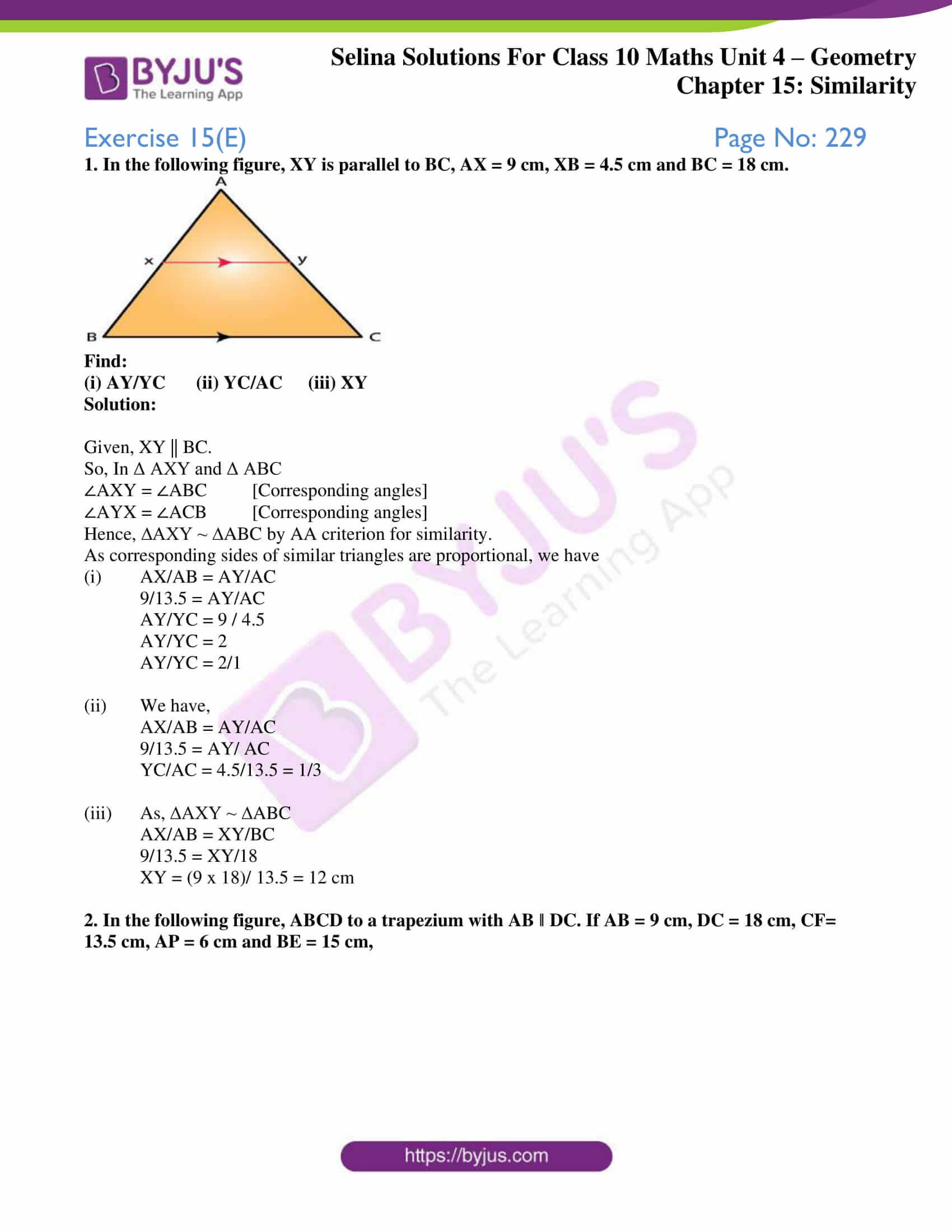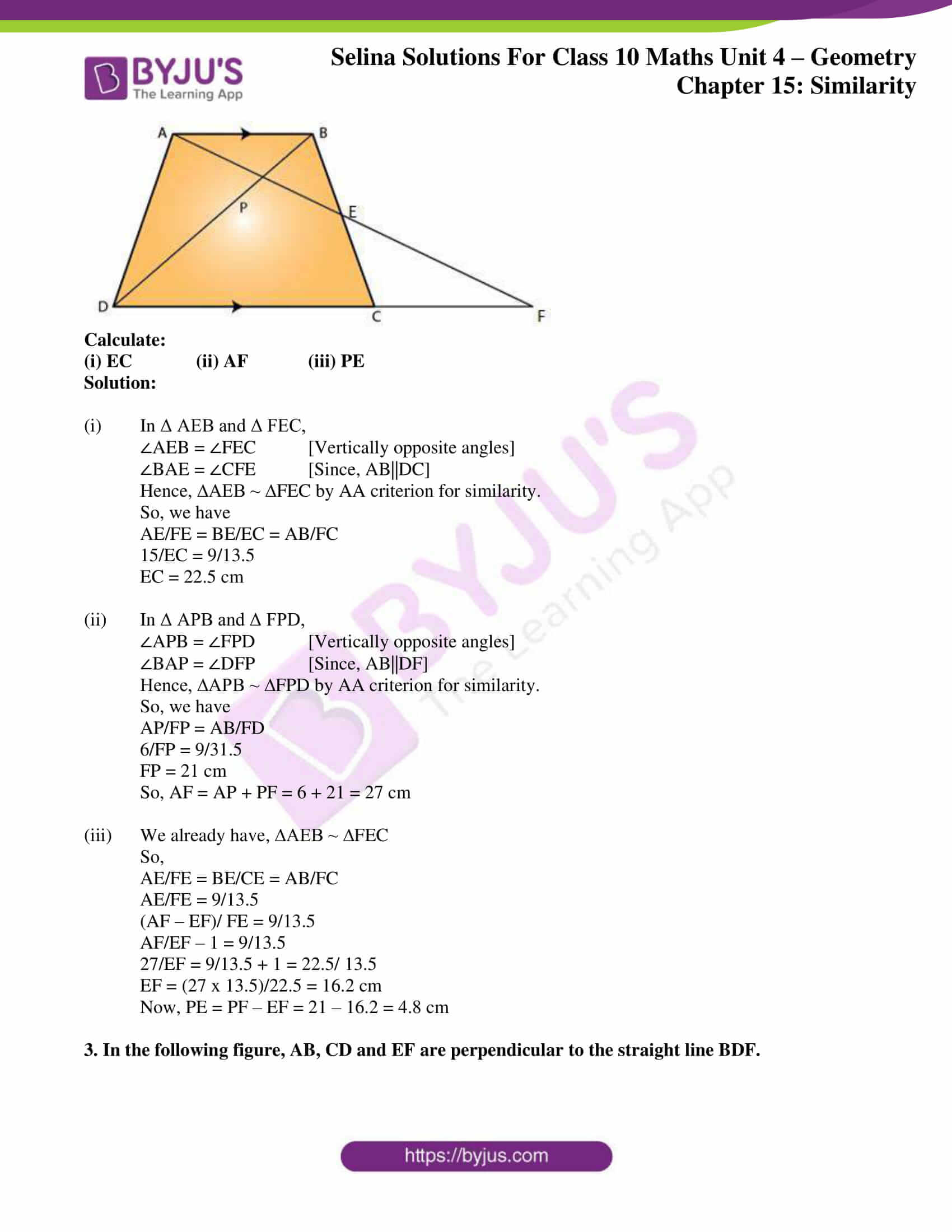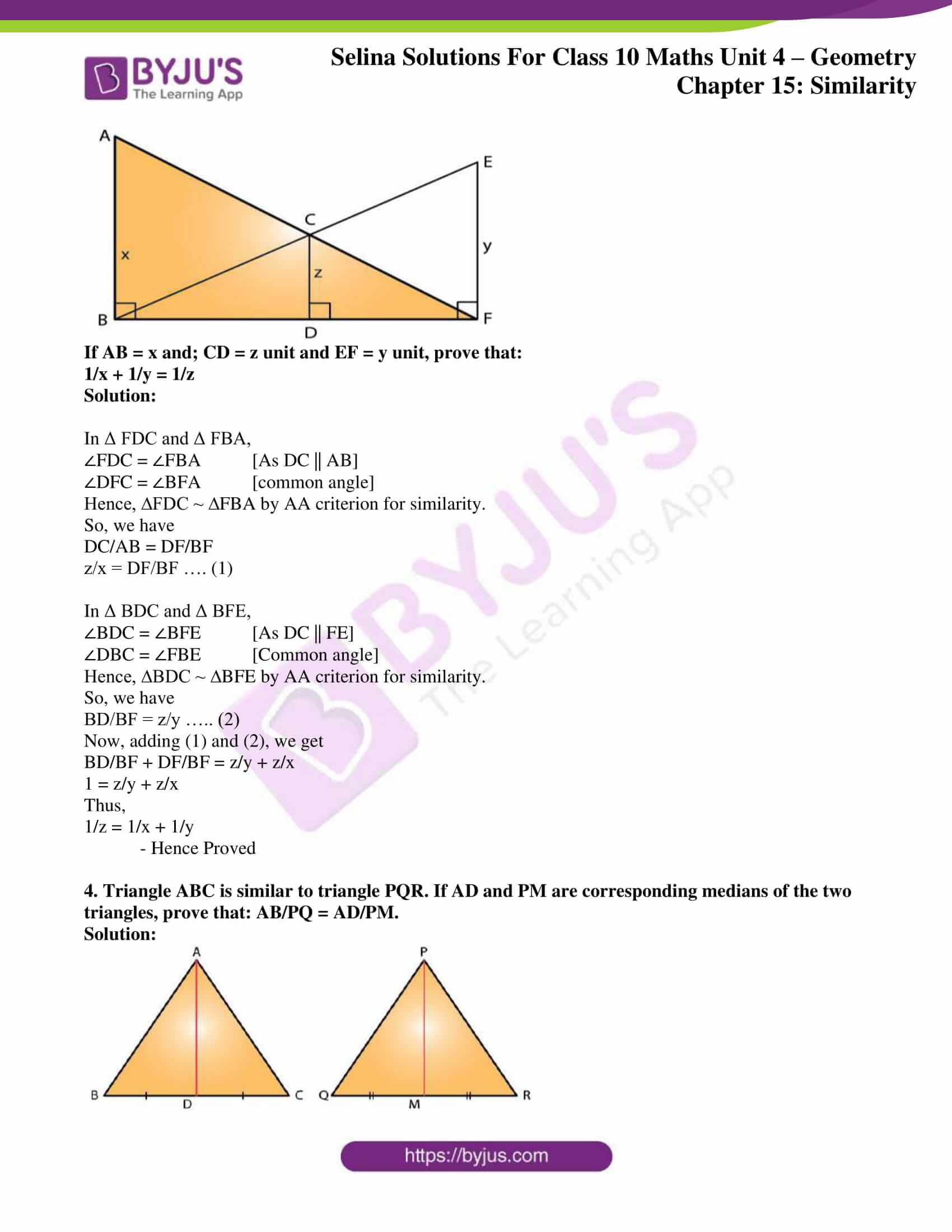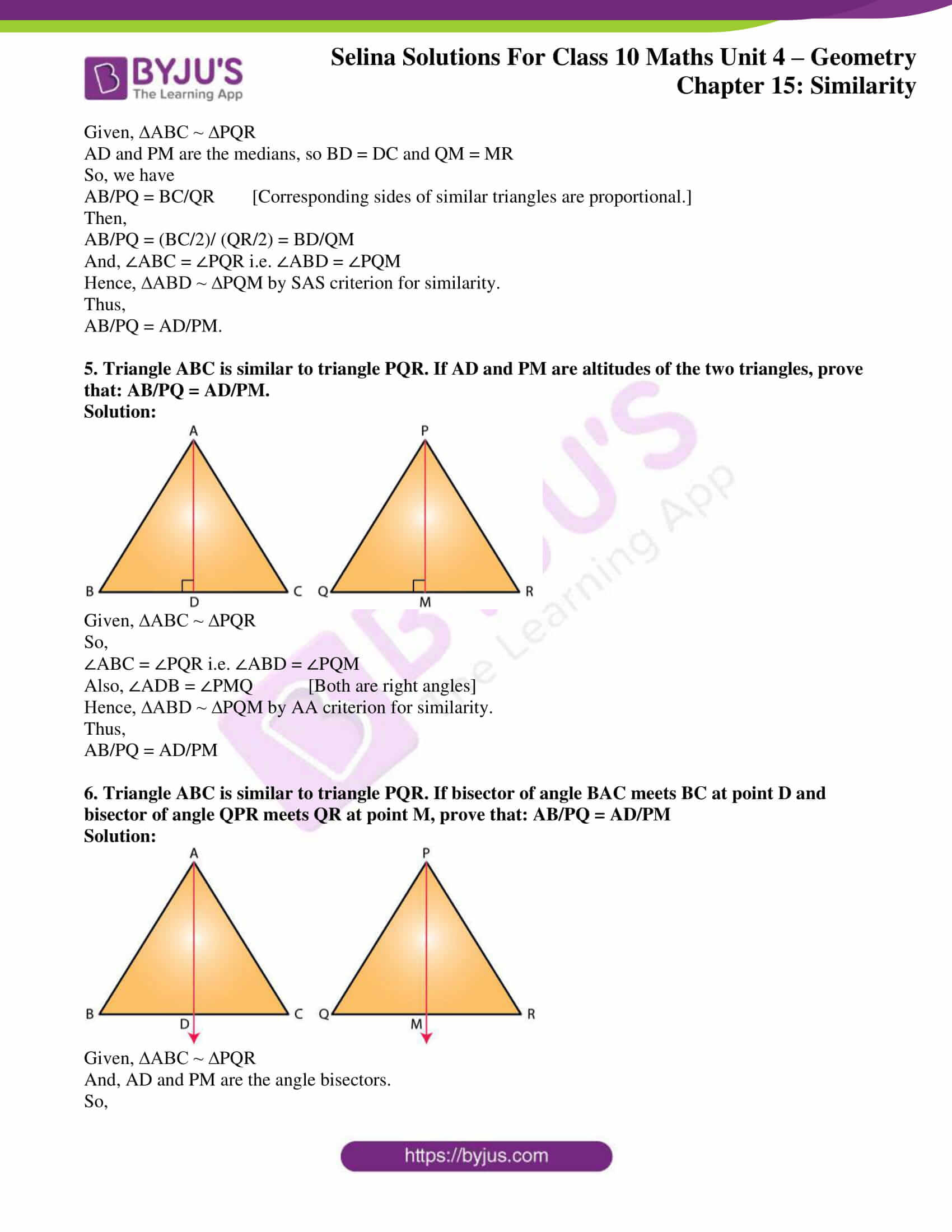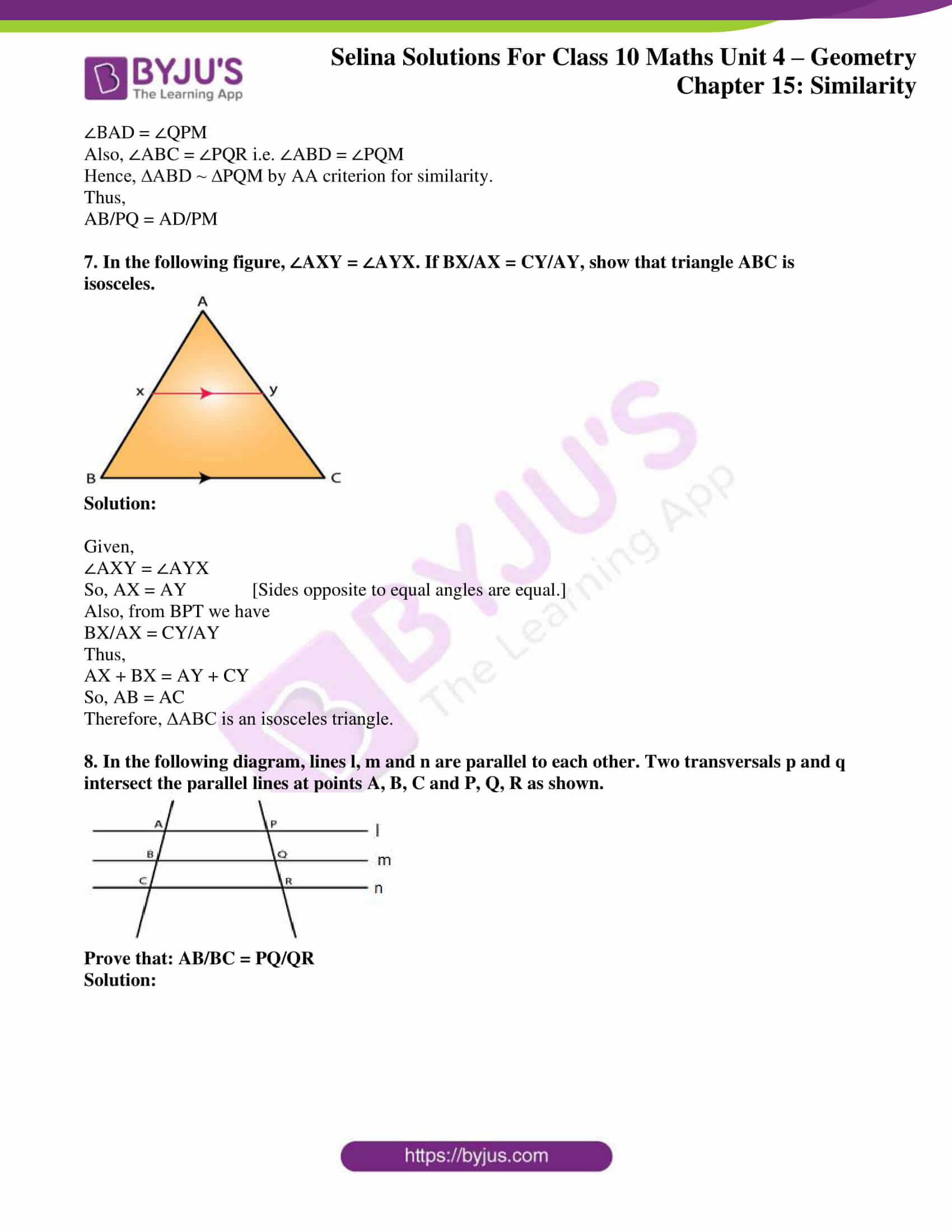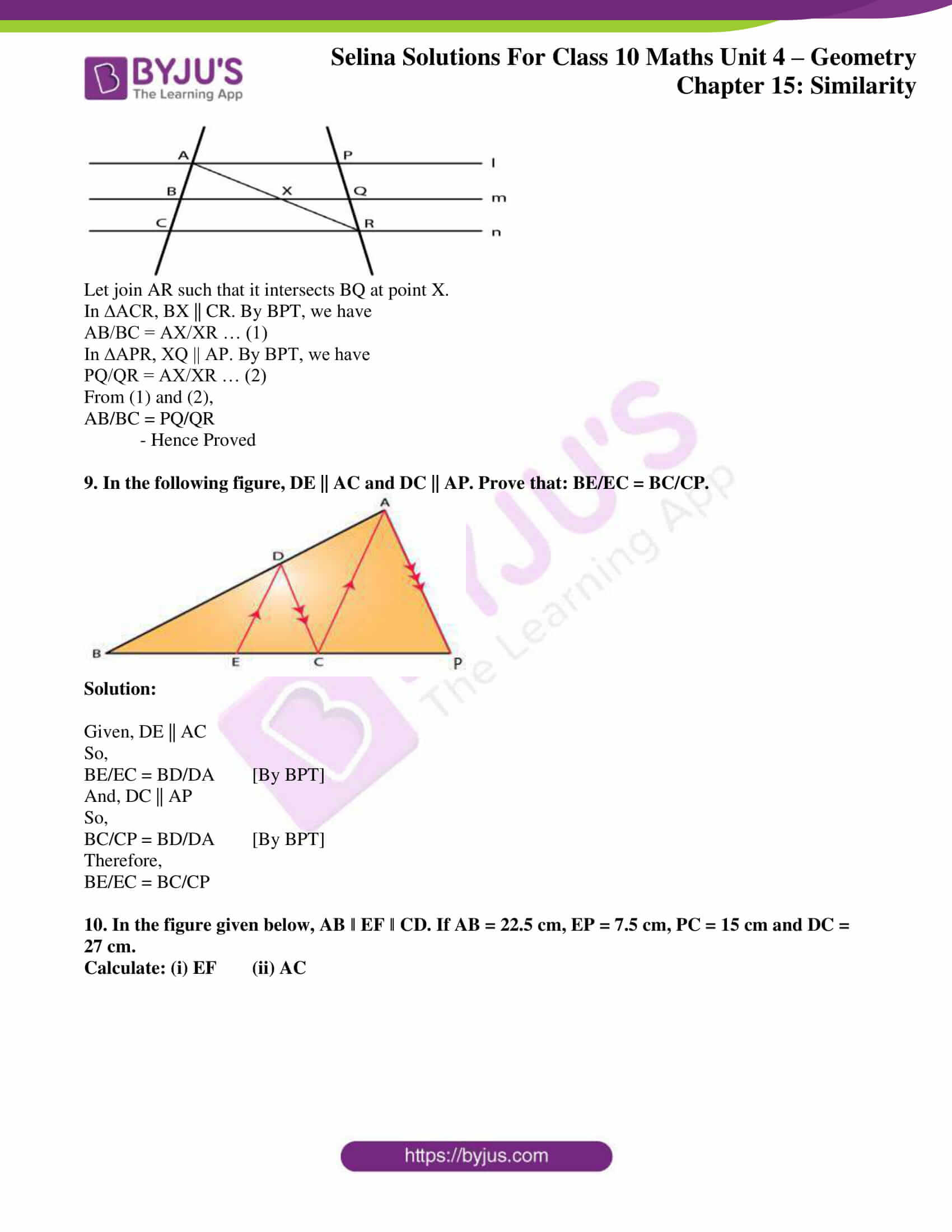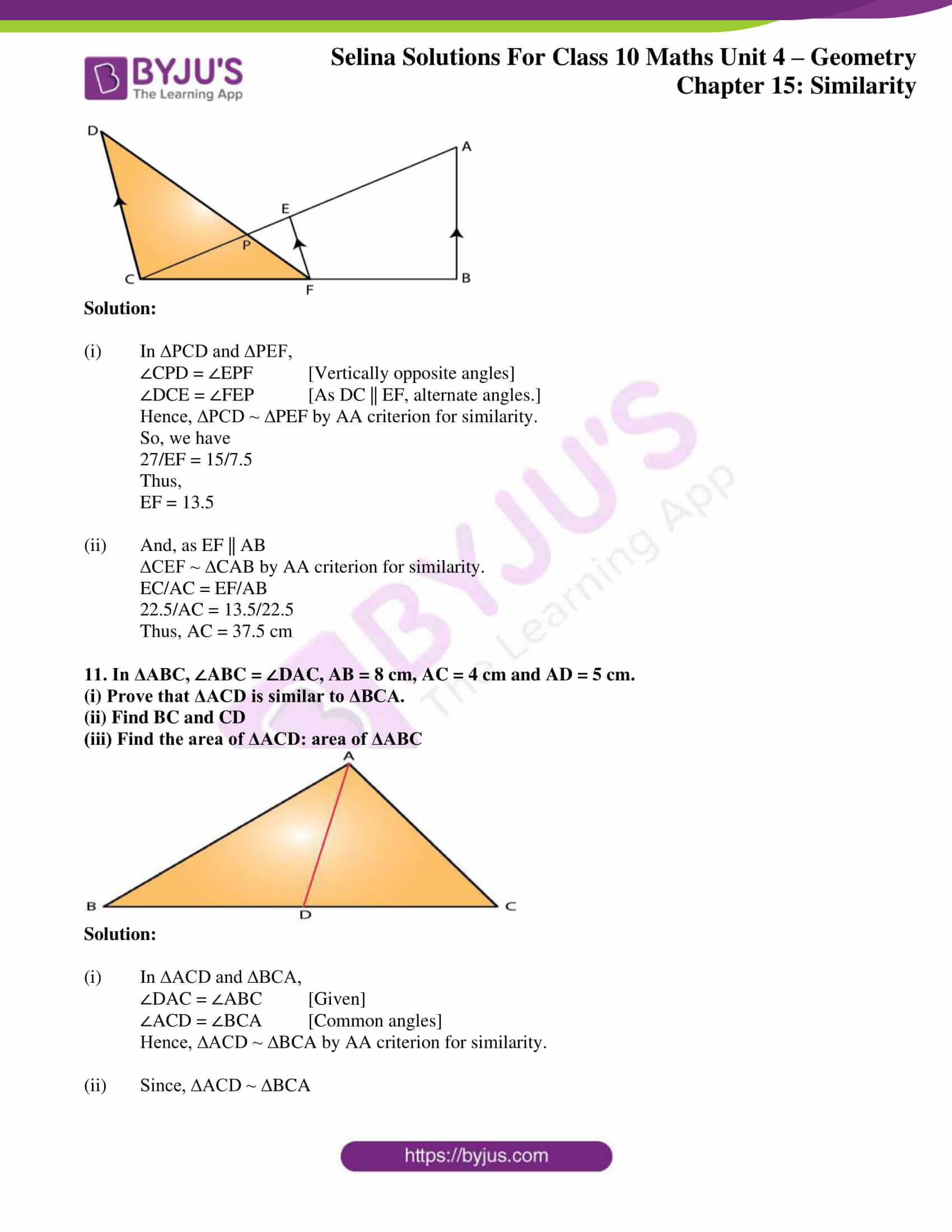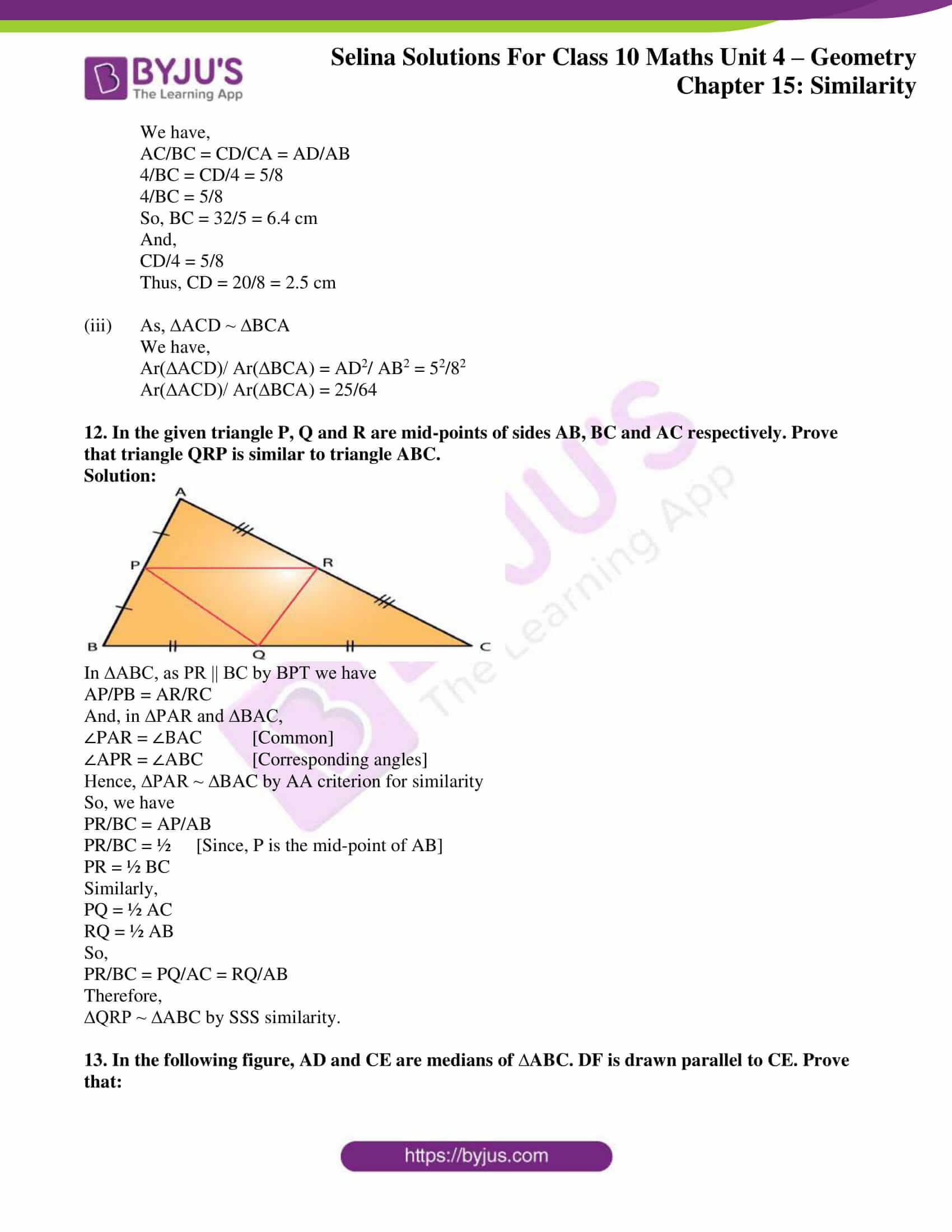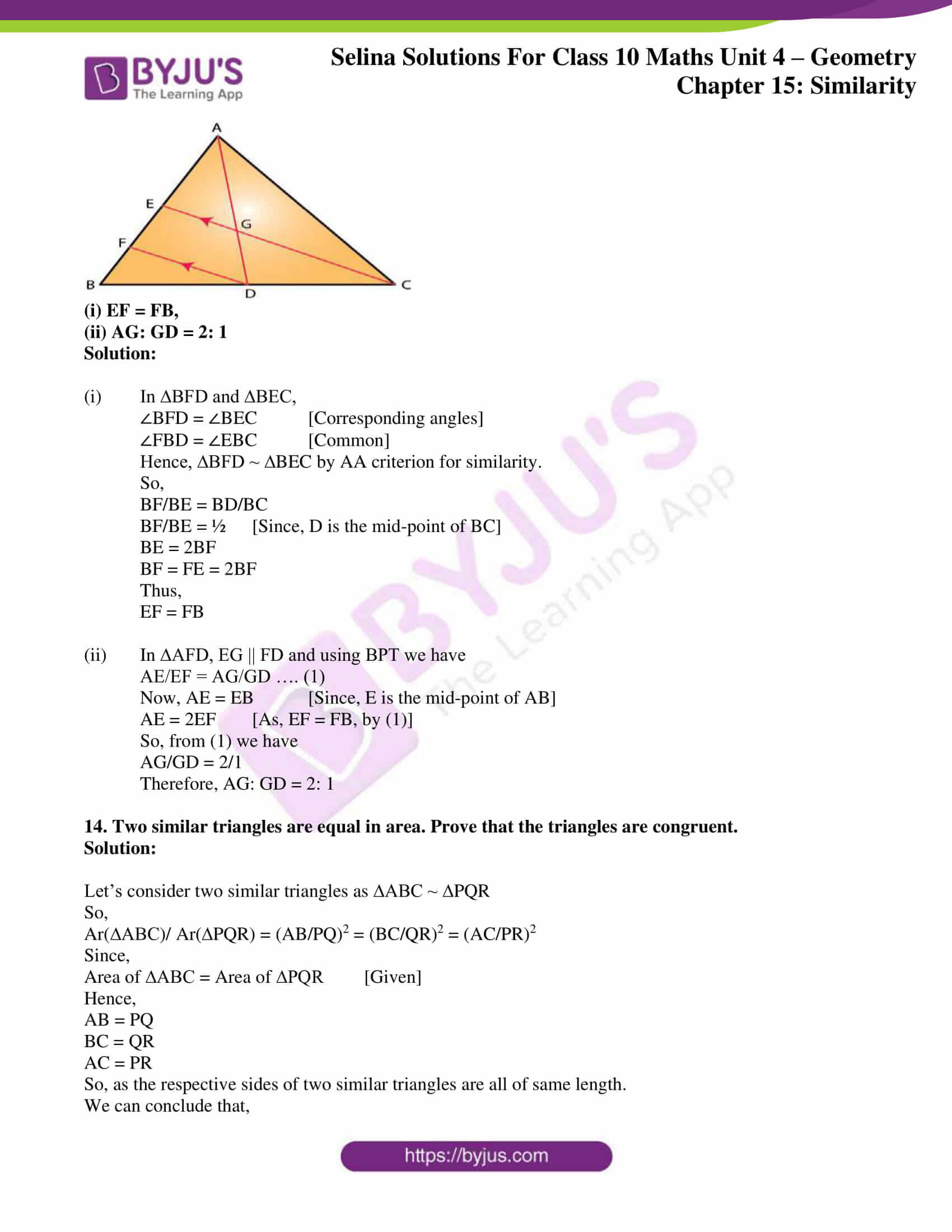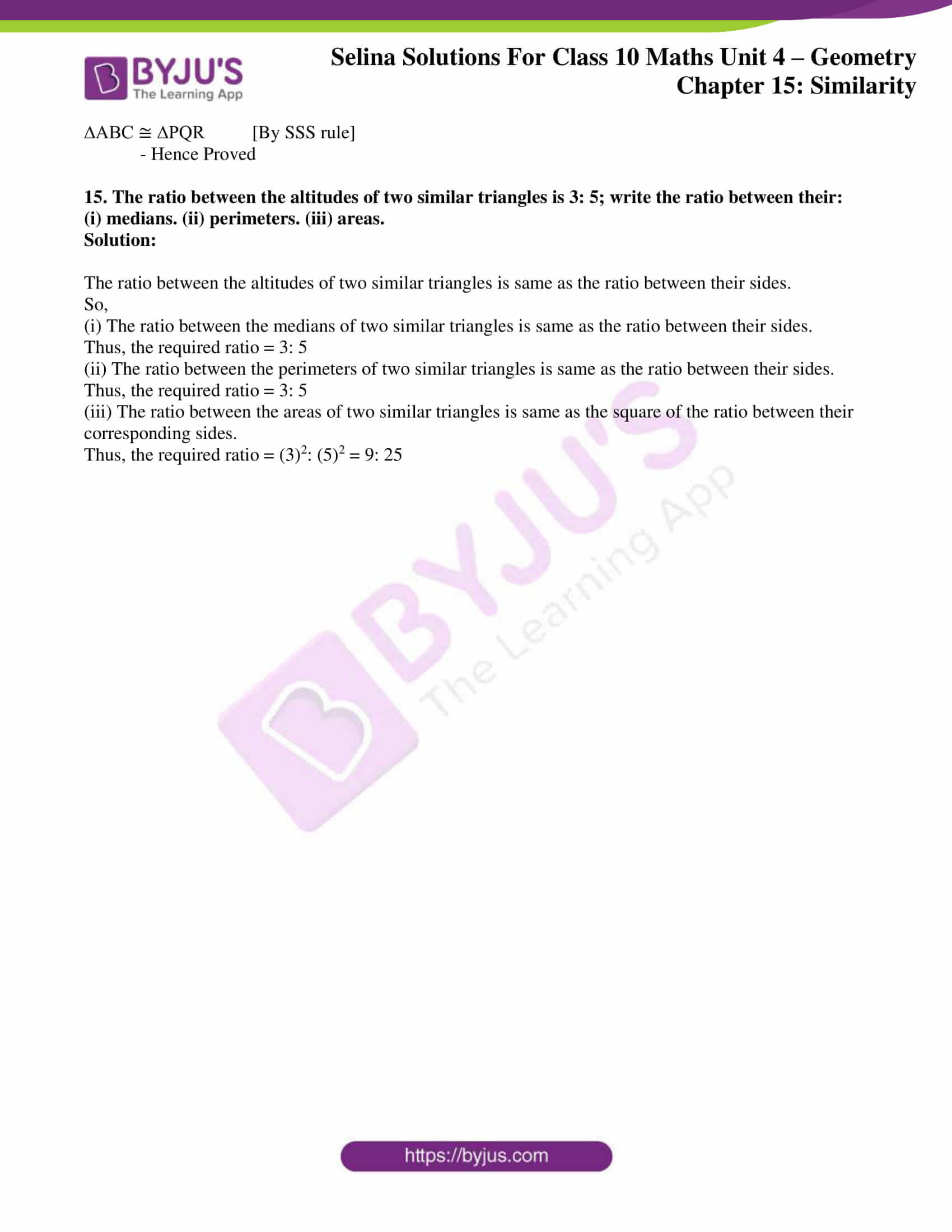### Access other exercises of Selina Solutions Concise Maths Class 10 Chapter 15 Similarity (With Applications to Maps and Models)

Exercise 15(A) Solutions

Exercise 15(B) Solutions

Exercise 15(C) Solutions

Exercise 15(D) Solutions

## Access Selina Solutions Concise Maths Class 10 Chapter 15 Similarity (With Applications to Maps and Models)

#### Exercise 15(E) Page No: 229

1. In the following figure, XY is parallel to BC, AX = 9 cm, XB = 4.5 cm and BC = 18 cm.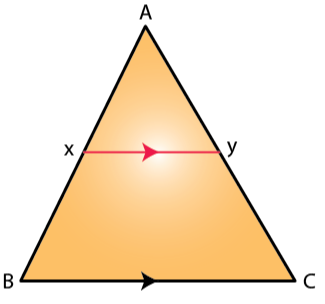Find:

(i) AY/YC (ii) YC/AC (iii) XY

Solution:

Given, XY || BC.

So, In Δ AXY and Δ ABC

∠AXY = ∠ABC [Corresponding angles]

∠AYX = ∠ACB [Corresponding angles]

Hence, ∆AXY ~ ∆ABC by AA criterion for similarity.

As corresponding sides of similar triangles are proportional, we have

(i) AX/AB = AY/AC

9/13.5 = AY/AC

AY/YC = 9 / 4.5

AY/YC = 2

AY/YC = 2/1

(ii) We have,

AX/AB = AY/AC

9/13.5 = AY/ AC

YC/AC = 4.5/13.5 = 1/3

(iii) As, ∆AXY ~ ∆ABC

AX/AB = XY/BC

9/13.5 = XY/18

XY = (9 x 18)/ 13.5 = 12 cm

2. In the following figure, ABCD to a trapezium with AB ‖ DC. If AB = 9 cm, DC = 18 cm, CF= 13.5 cm, AP = 6 cm and BE = 15 cm,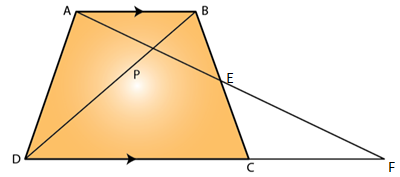Calculate:

(i) EC (ii) AF (iii) PE

Solution:

(i) In Δ AEB and Δ FEC,

∠AEB = ∠FEC [Vertically opposite angles]

∠BAE = ∠CFE [Since, AB||DC]

Hence, ∆AEB ~ ∆FEC by AA criterion for similarity.

So, we have

AE/FE = BE/EC = AB/FC

15/EC = 9/13.5

EC = 22.5 cm

(ii) In Δ APB and Δ FPD,

∠APB = ∠FPD [Vertically opposite angles]

∠BAP = ∠DFP [Since, AB||DF]

Hence, ∆APB ~ ∆FPD by AA criterion for similarity.

So, we have

AP/FP = AB/FD

6/FP = 9/31.5

FP = 21 cm

So, AF = AP + PF = 6 + 21 = 27 cm

(iii) We already have, ∆AEB ~ ∆FEC

So,

AE/FE = BE/CE = AB/FC

AE/FE = 9/13.5

(AF – EF)/ FE = 9/13.5

AF/EF – 1 = 9/13.5

27/EF = 9/13.5 + 1 = 22.5/ 13.5

EF = (27 x 13.5)/22.5 = 16.2 cm

Now, PE = PF – EF = 21 – 16.2 = 4.8 cm

3. In the following figure, AB, CD and EF are perpendicular to the straight line BDF.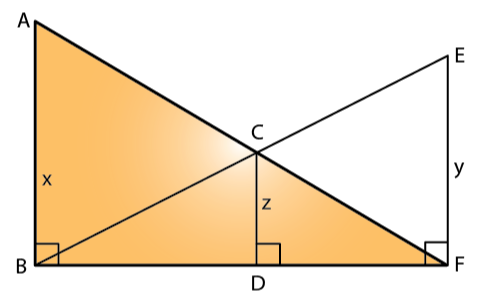If AB = x and; CD = z unit and EF = y unit, prove that:

1/x + 1/y = 1/z

Solution:

In Δ FDC and Δ FBA,

∠FDC = ∠FBA [As DC || AB]

∠DFC = ∠BFA [common angle]

Hence, ∆FDC ~ ∆FBA by AA criterion for similarity.

So, we have

DC/AB = DF/BF

z/x = DF/BF …. (1)

In Δ BDC and Δ BFE,

∠BDC = ∠BFE [As DC || FE]

∠DBC = ∠FBE [Common angle]

Hence, ∆BDC ~ ∆BFE by AA criterion for similarity.

So, we have

BD/BF = z/y ….. (2)

Now, adding (1) and (2), we get

BD/BF + DF/BF = z/y + z/x

1 = z/y + z/x

Thus,

1/z = 1/x + 1/y

– Hence Proved

4. Triangle ABC is similar to triangle PQR. If AD and PM are corresponding medians of the two triangles, prove that: AB/PQ = AD/PM.

Solution: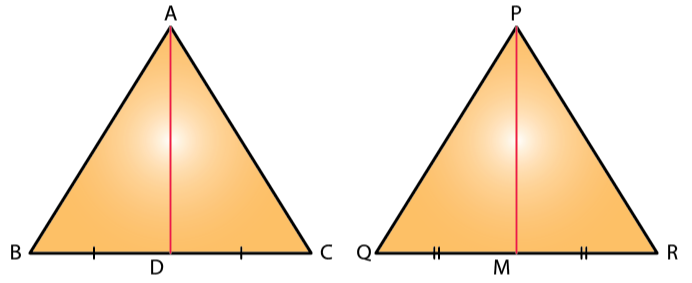Given, ∆ABC ~ ∆PQR

AD and PM are the medians, so BD = DC and QM = MR

So, we have

AB/PQ = BC/QR [Corresponding sides of similar triangles are proportional.]

Then,

AB/PQ = (BC/2)/ (QR/2) = BD/QM

And, ∠ABC = ∠PQR i.e. ∠ABD = ∠PQM

Hence, ∆ABD ~ ∆PQM by SAS criterion for similarity.

Thus,

5. Triangle ABC is similar to triangle PQR. If AD and PM are altitudes of the two triangles, prove that: AB/PQ = AD/PM.

Solution: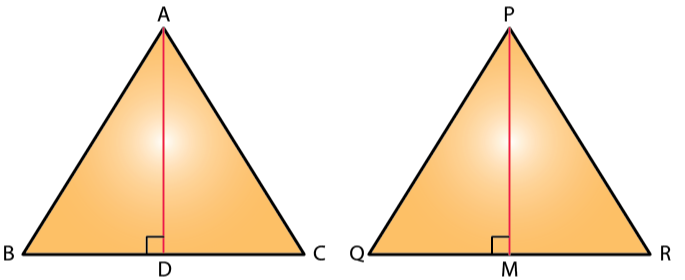Given, ∆ABC ~ ∆PQR

So,

∠ABC = ∠PQR i.e. ∠ABD = ∠PQM

Also, ∠ADB = ∠PMQ [Both are right angles]

Hence, ∆ABD ~ ∆PQM by AA criterion for similarity.

Thus,

6. Triangle ABC is similar to triangle PQR. If bisector of angle BAC meets BC at point D and bisector of angle QPR meets QR at point M, prove that: AB/PQ = AD/PM

Solution: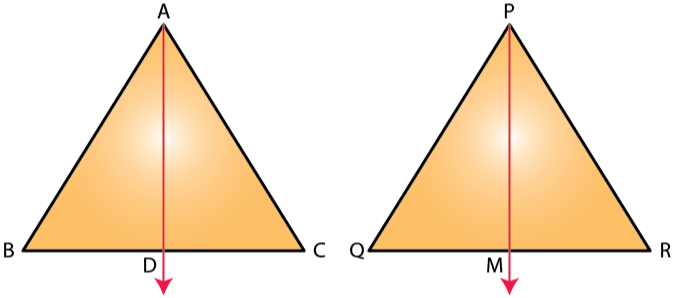Given, ∆ABC ~ ∆PQR

And, AD and PM are the angle bisectors.

So,

Also, ∠ABC = ∠PQR i.e. ∠ABD = ∠PQM

Hence, ∆ABD ~ ∆PQM by AA criterion for similarity.

Thus,

7. In the following figure, ∠AXY = ∠AYX. If BX/AX = CY/AY, show that triangle ABC is isosceles.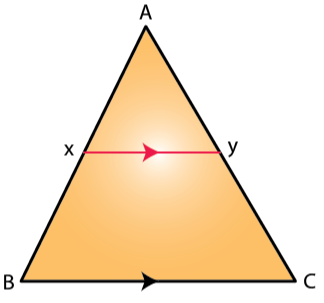Solution:

Given,

∠AXY = ∠AYX

So, AX = AY [Sides opposite to equal angles are equal.]

Also, from BPT we have

BX/AX = CY/AY

Thus,

AX + BX = AY + CY

So, AB = AC

Therefore, ∆ABC is an isosceles triangle.

8. In the following diagram, lines l, m and n are parallel to each other. Two transversals p and q intersect the parallel lines at points A, B, C and P, Q, R as shown.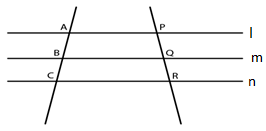Prove that: AB/BC = PQ/QR

Solution: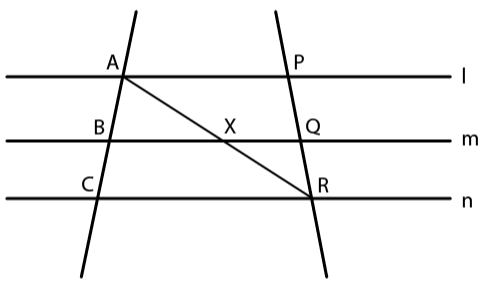Let join AR such that it intersects BQ at point X.

In ∆ACR, BX || CR. By BPT, we have

AB/BC = AX/XR … (1)

In ∆APR, XQ || AP. By BPT, we have

PQ/QR = AX/XR … (2)

From (1) and (2),

AB/BC = PQ/QR

– Hence Proved

9. In the following figure, DE || AC and DC || AP. Prove that: BE/EC = BC/CP.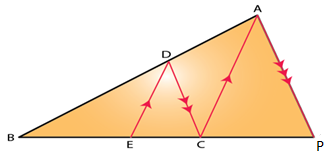Solution:

Given, DE || AC

So,

BE/EC = BD/DA [By BPT]

And, DC || AP

So,

BC/CP = BD/DA [By BPT]

Therefore,

BE/EC = BC/CP

10. In the figure given below, AB ‖ EF ‖ CD. If AB = 22.5 cm, EP = 7.5 cm, PC = 15 cm and DC = 27 cm.

Calculate: (i) EF (ii) AC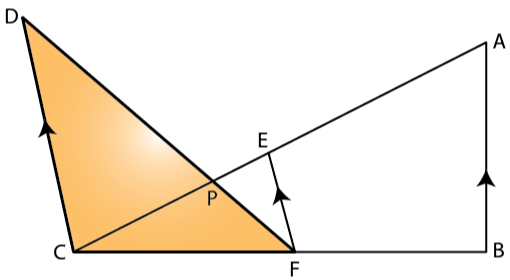Solution:

(i) In ∆PCD and ∆PEF,

∠CPD = ∠EPF [Vertically opposite angles]

∠DCE = ∠FEP [As DC || EF, alternate angles.]

Hence, ∆PCD ~ ∆PEF by AA criterion for similarity.

So, we have

27/EF = 15/7.5

Thus,

EF = 13.5

(ii) And, as EF || AB

∆CEF ~ ∆CAB by AA criterion for similarity.

EC/AC = EF/AB

22.5/AC = 13.5/22.5

Thus, AC = 37.5 cm

11. In ΔABC, ∠ABC = ∠DAC, AB = 8 cm, AC = 4 cm and AD = 5 cm.

(i) Prove that ΔACD is similar to ΔBCA.

(ii) Find BC and CD

(iii) Find the area of ΔACD: area of ΔABC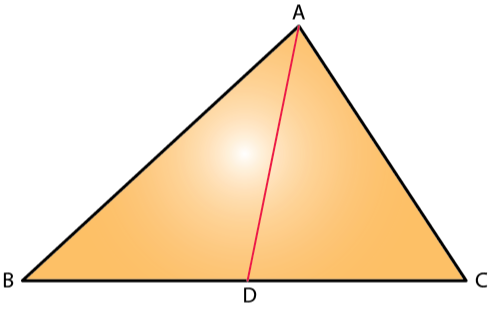Solution:

(i) In ∆ACD and ∆BCA,

∠DAC = ∠ABC [Given]

∠ACD = ∠BCA [Common angles]

Hence, ∆ACD ~ ∆BCA by AA criterion for similarity.

(ii) Since, ∆ACD ~ ∆BCA

We have,

4/BC = CD/4 = 5/8

4/BC = 5/8

So, BC = 32/5 = 6.4 cm

And,

CD/4 = 5/8

Thus, CD = 20/8 = 2.5 cm

(iii) As, ∆ACD ~ ∆BCA

We have,

Ar(∆ACD)/ Ar(∆BCA) = AD2/ AB2 = 52/82

Ar(∆ACD)/ Ar(∆BCA) = 25/64

12. In the given triangle P, Q and R are mid-points of sides AB, BC and AC respectively. Prove that triangle QRP is similar to triangle ABC.

Solution: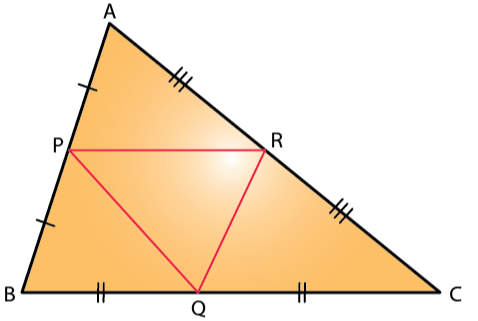In ∆ABC, as PR || BC by BPT we have

AP/PB = AR/RC

And, in ∆PAR and ∆BAC,

∠PAR = ∠BAC [Common]

∠APR = ∠ABC [Corresponding angles]

Hence, ∆PAR ~ ∆BAC by AA criterion for similarity

So, we have

PR/BC = AP/AB

PR/BC = ½ [Since, P is the mid-point of AB]

PR = ½ BC

Similarly,

PQ = ½ AC

RQ = ½ AB

So,

PR/BC = PQ/AC = RQ/AB

Therefore,

∆QRP ~ ∆ABC by SSS similarity.

13. In the following figure, AD and CE are medians of ∆ABC. DF is drawn parallel to CE. Prove that: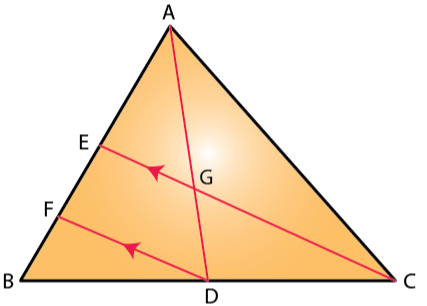(i) EF = FB,

(ii) AG: GD = 2: 1

Solution:

(i) In ∆BFD and ∆BEC,

∠BFD = ∠BEC [Corresponding angles]

∠FBD = ∠EBC [Common]

Hence, ∆BFD ~ ∆BEC by AA criterion for similarity.

So,

BF/BE = BD/BC

BF/BE = ½ [Since, D is the mid-point of BC]

BE = 2BF

BF = FE = 2BF

Thus,

EF = FB

(ii) In ∆AFD, EG || FD and using BPT we have

AE/EF = AG/GD …. (1)

Now, AE = EB [Since, E is the mid-point of AB]

AE = 2EF [As, EF = FB, by (1)]

So, from (1) we have

AG/GD = 2/1

Therefore, AG: GD = 2: 1

14. Two similar triangles are equal in area. Prove that the triangles are congruent.

Solution:

Let’s consider two similar triangles as ∆ABC ~ ∆PQR

So,

Ar(∆ABC)/ Ar(∆PQR) = (AB/PQ)2 = (BC/QR)2 = (AC/PR)2

Since,

Area of ∆ABC = Area of ∆PQR [Given]

Hence,

AB = PQ

BC = QR

AC = PR

So, as the respective sides of two similar triangles are all of same length.

We can conclude that,

∆ABC ≅ ∆PQR [By SSS rule]

– Hence Proved

15. The ratio between the altitudes of two similar triangles is 3: 5; write the ratio between their:

(i) medians. (ii) perimeters. (iii) areas.

Solution:

The ratio between the altitudes of two similar triangles is same as the ratio between their sides.

So,

(i) The ratio between the medians of two similar triangles is same as the ratio between their sides.

Thus, the required ratio = 3: 5

(ii) The ratio between the perimeters of two similar triangles is same as the ratio between their sides.

Thus, the required ratio = 3: 5

(iii) The ratio between the areas of two similar triangles is same as the square of the ratio between their corresponding sides.

Thus, the required ratio = (3)2: (5)2 = 9: 25